# Multi-Step Measurement Conversions

in Education2 years ago

Hi there. In this math post, I would like to cover multi-step measurement conversions. It is assumed that the reader is comfortable with multiplication and division.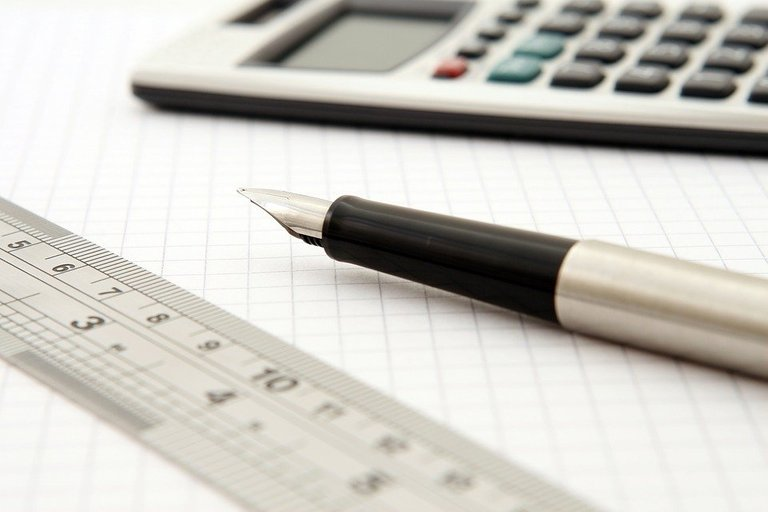Pixabay Image Source

## Topics

• Some Common Measurement Conversions
• Examples Of Multi-Step Measurement Conversions
• Practice Problems
• Solutions To Practice Problems

## Some Measurement Conversions

Measurement conversions are used for comparing measurement quantities and for math calculations when it comes to area, perimeter, volume, etc.

Here are some measurement conversions.

Time

• 1 Hour = 60 Minutes
• 1 Minute = 60 Seconds
• 1 Week = 7 Days
• A year has 365 days with one extra day on a leap year.
• There are 12 months in a year.
• In a day, there are 24 hours.

Distance

• 1 Centimetre (cm) = 10 Millimetres (mm)
• 1 Decimetre (dm) = 10 Centimetres
• 1 Kilometre (km) = 1000 Metres
• One metre is 100 centimetres.
• There are 12 inches in one foot.
• One yard is equal to 36 inches.

Volume

• There are 1000 millilitres (mL) in one litre.
• One gallon contains 8 pints.
• One quart is 2 pints.
• One litre is 33.81 (US) fluid ounces.

Mass & Weight

• One Kilogram (kg) = 1000 grams
• One Gram is 1000 milligrams
• One Pound is about 2.2kg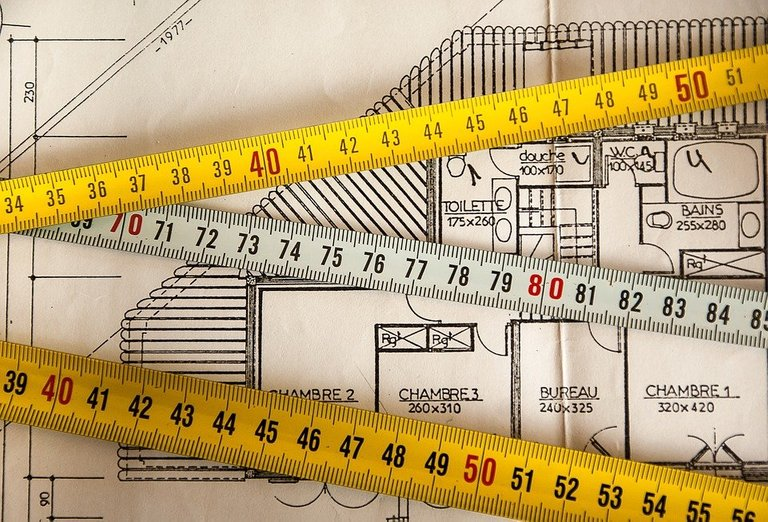Pixabay Image Source

## Examples Of Multi-Step Measurement Conversions

When it comes to multi-step measurement conversions, it involves a lot of multiplication and division of large numbers. Let's look at some examples.

Example One

How many centimetres are in 2 kilometres?

Instead of going from kilometres into centimetres straight away, it is easier to go from kilometres to metres and then from metres to centimetres. One kilometre is 1000 metres so two kilometeres would be 2000 metres.

`2 km = 2000m`

It is also known that one metre is 100 centimetres. Multiply the 2000 metres by 100 centimetres per metre.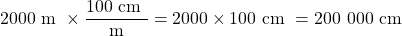There are two hundred thousand centimetres in 2 kilometres.

Example Two

How many minutes are there in a day?

It is known that there are 24 hours in one day. Each hour has 60 minutes. Multiply the 24 hours by 60 minutes in each hour.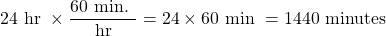Example Three

How many milligrams are there in one kilogram?

One kilogram is equal to one thousand grams. In each gram there are 1000 milligrams.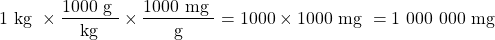In one kilogram, there are one million milligrams.

Example Four

How many fluid ounces are there in 7000 millilitres?

There are 1000 millilitres (mL) in one litre. In addition, there are 33.81 (US) fluid ounces in each litre.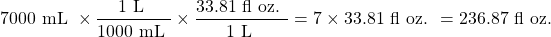Pixabay Image Source

## Practice Problems

1. How many hours are there in two weeks?

2. Convert ten thousand centimetres to kilometres.

3. How many pounds are there in 2000 grams?

4. How many minutes are there in a non-leap year?

5. The book Outliers by Malcolm Gladwell mentions the 10000 hour rule for being world-class at something. How many days are there in 10000 hours? How many weeks are there in 10000 hours?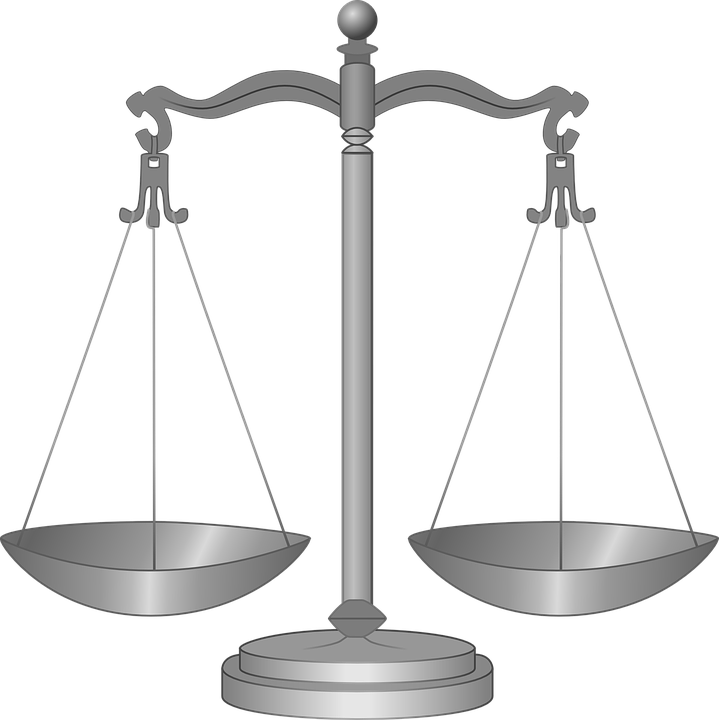Pixabay Image Source

## Solutions To Practice Problems

Question One

There are 7 days in one week. In each day there are 24 hours. The calculation would be `2 x 7 x 24 = 336`. In two weeks, there are 336 hours.

Question Two

One hundred centimetres is one metre. This makes 10000 cm into 100 metres. Every 1000 metres is one kilometre. Dividing 100 by one thousand gives 0.1km. Ten thousand centimetres is equal to one tenth of a kilometre.

Question Three

Two thousand grams is equal to 2 kilograms. One pound is equal to 2.2 kilograms. Dividing 2 kilograms by 2.2 kg per pound (11/5) gives `10/11` or a pound or about 0.9090...

Question Four

A non-leap year has 365 days. Each day has 24 hours and each hour has 60 minutes. Multiply 365 by 24 and by 60 to obtain 525,600 minutes in a year.

Question Five

Ten thousand hours is equal to 416 full days with 16 hours left over. One week is seven days or about 168 hours.

Dividing 10000 hours by 168 hours gives 59 weeks with 88 hours left over. This 88 hours left over is 3 2/3 days.Pixabay Image Source